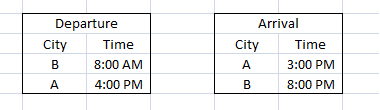# CAT 2007

Instructions

Directions for the following four questions: Each question is followed by two statements A and B. Indicate your responses based on data sufficiency

Question 1

The average weight of a class of 100 students is 45 kg. The class consists of two sections, I and II, each with 50 students. The average weight, $$W_I$$ , of Section I is smaller than the average weight, $$W_{II}$$ , of Section II. If the heaviest student, say Deepak, of Section II is moved to Section I, and the lightest student, say Poonam, of Section I is moved to Section II, then the average weights of the two sections are switched, i.e., the average weight of Section I becomes $$W_{II}$$ and that of Section II becomes $$W_I$$ . What is the weight of Poonam?

A: $$W_{II} - W_I = 1.0$$

B: Moving Deepak from Section II to I (without any move from I to II) makes the average weights of the two sections equal.

Question 2

ABC Corporation is required to maintain at least 400 Kilolitres of water at all times in its factory, in order to meet safety and regulatory requirements. ABC is considering the suitability of a spherical tank with uniform wall thickness for the purpose. The outer diameter of the tank is 10 meters. Is the tank capacity adequate to meet ABC’s requirements?

A: The inner diameter of the tank is at least 8 meters.

B: The tank weighs 30,000 kg when empty, and is made of a material with density of 3 gm/cc.

Question 3

Consider integers x, y and z. What is the minimum possible value of $$x^2 + y^2 + z^2$$?

A: x + y + z = 89

B: Among x, y, z two are equal.

Question 4

Rahim plans to draw a square JKLM with a point O on the side JK but is not successful. Why is Rahim unable to draw the square?

A: The length of OM is twice that of OL.

B: The length of OM is 4 cm

Instructions

Directions for the following two questions: Cities A and B are in different time zones. A is located 3000 km east of B. The table below describes the schedule of an airline operating non-stop flights between A and B. All the times indicated are local and on the same day.

Assume that planes cruise at the same speed in both directions. However, the effective speed is influenced by a steady wind blowing from east to west at 50 km per hour.Question 5

What is the time difference between A and B?

Question 6

What is the plane’s cruising speed in km per hour?

Instructions

Directions for the following two questions: Shabnam is considering three alternatives to invest her surplus cash for a week. She wishes to guarantee maximum returns on her investment. She has three options, each of which can be utilized fully or partially in conjunction with others.

Option A: Invest in a public sector bank. It promises a return of +0.10%.

Option B: Invest in mutual funds of ABC Ltd. A rise in the stock market will result in a return of +5%, while a fall will entail a return of – 3%.

Option C: Invest in mutual funds of CBA Ltd. A rise in the stock market will result in a return of – 2.5%, while a fall will entail a return of + 2%.

Question 7

Question 8

Instructions

Directions for the following two questions:

Let S be the set of all pairs (i, j) where 1 <= i < j <= n , and n >= 4 (i and j are natural numbers). Any two distinct members of S are called “friends” if they have one constituent of the pairs in common and “enemies” otherwise.

For example, if n = 4, then S = {(1, 2), (1, 3), (1, 4), (2, 3), (2, 4), (3, 4)}. Here, (1, 2) and (1, 3) are friends, (1,2) and (2, 3) are also friends, but (1,4) and (2, 3) are enemies.

Question 9

For general n, how many enemies will each member of S have?

Question 10

For general n, consider any two members of S that are friends. How many other members of S will be common friends of both these members?

OR